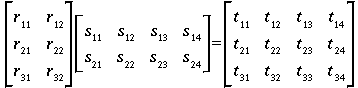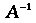ECE 417 - Introduction to Robotics
Notes

Matrix review:

Multiplication:where(dot product)

sizes: 3x2 times 2x4 = 3x4

Remember: Row, Column (RC)

Note: Matrix multiplication does not commute:

In generalBut associate property holds:For many matrices(i.e., non-singular matrices) there exists an inversesuch thatWhere I is an identity matrix. E.g.,Matrix transpose:interchanges rows and columns